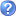• Molecular Expressions
Electricity and Magnetism - Interactive Java Tutorials: Resistance at the Molecular Level; EXCELLENT visual treatment of electrons flowing through a filament and atoms, as well as other electrons, resisting the flow.
• The Blobz Guide to Electric Circuits
Interactive site for learning about electricity. This is a TERRIFIC way for students to learn and manipulate components of a circuit and learn to draw diagrams.
by 2 users
• Electricity
Interactive Science Revision. Lots of digital animations and interactives that teach students about electricity concepts, e.g., circuits, conductors, insulators, resistors, voltage
• National High Magnetic Field Laboratory - Current Flow Tutorial
Current Flow This tutorial illustrates how the flow of water through a system of pipes can be used to understand the flow of current through an electric circuit. Here blue particles represent the flow of water, moving as electrons do through a circuit to create electricity. The rate of water flow, defined as the volume of the fluid moving past a certain point in a fixed amount of time, is comparable to the rate of charge passing a point in a circuit (electric current), which is measured in amperes (coulombs/second).
• BBC - Schools Science Clips - Changing circuits
• Concentration Game for Electrical Symbols
• eChalk
• Electricity
Circuits, Conductors, and Batteries | eThemes | eMINTS